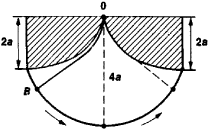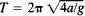# Cycloidal Pendulum

## cycloidal pendulum

[sī′klȯid·əl ′pen·jə·ləm]
(mechanics)
A modification of a simple pendulum in which a weight is suspended from a cord which is slung between two pieces of metal shaped in the form of cycloids; as the bob swings, the cord wraps and unwraps on the cycloids; the pendulum has a period that is independent of the amplitude of the swing.

## Cycloidal Pendulum

a simple pendulum whose oscillations under the force of gravity describe an arc of a cycloid that has a vertical axis and is convex downward.Figure 1. A cycloidal pendulum

A cycloidal pendulum may be constructed by suspending a bob B on a string of length 4a and, as the bob oscillates, causing the string to wrap and unwrap itself about two pieces of metal having the shape of identical cycloids (the shaded regions in Figure 1) with a generating circle of radius a. The bob B will then describe the same cycloid; that is, it will be a cycloidal pendulum.

The period of oscillation of a cycloidal pendulum about the equilibrium position—that is, about the lowest point of the cycloid—is independent of the amplitude of the swing. The period is given by the equation, where g is the acceleration of gravity. Thus, the oscillations of a cycloidal pendulum are strictly isochronous. This property is characteristic of other pendulums only approximately and only for small oscillations.

Site: Follow: Share:
Open / Close